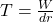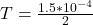## Calculate surface tension of an enlarged radius of 4cm to 6cm and amount of work necessary for enlargement was 1.5×10^-4 joules

Question

Calculate surface tension of an enlarged radius of 4cm to 6cm and amount of work necessary for enlargement was 1.5×10^-4 joules

in progress 0
2 months 2021-07-15T16:51:04+00:00 1 Answers 2 views 0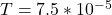Explanation:

From the question we are told that:

RadiusWork done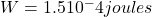Generally the equation for Work done is mathematically given by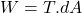Therefore